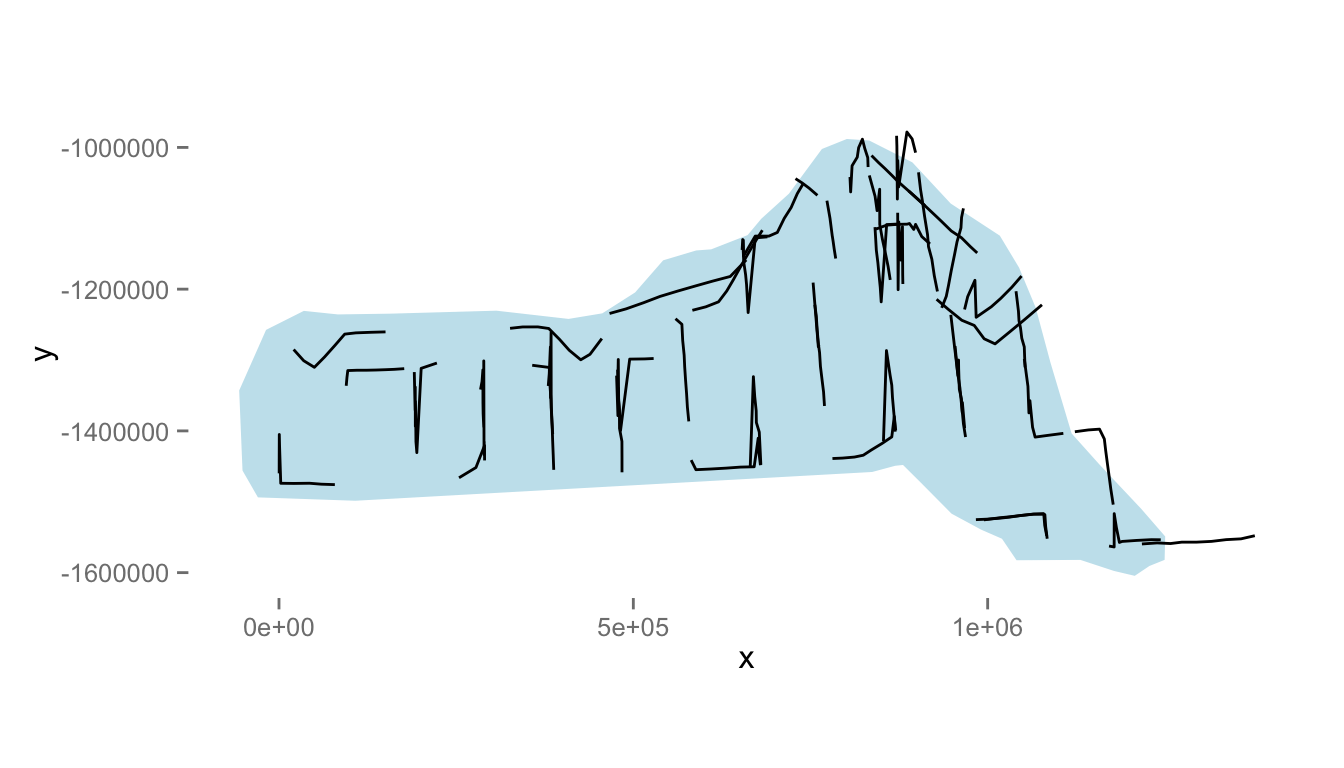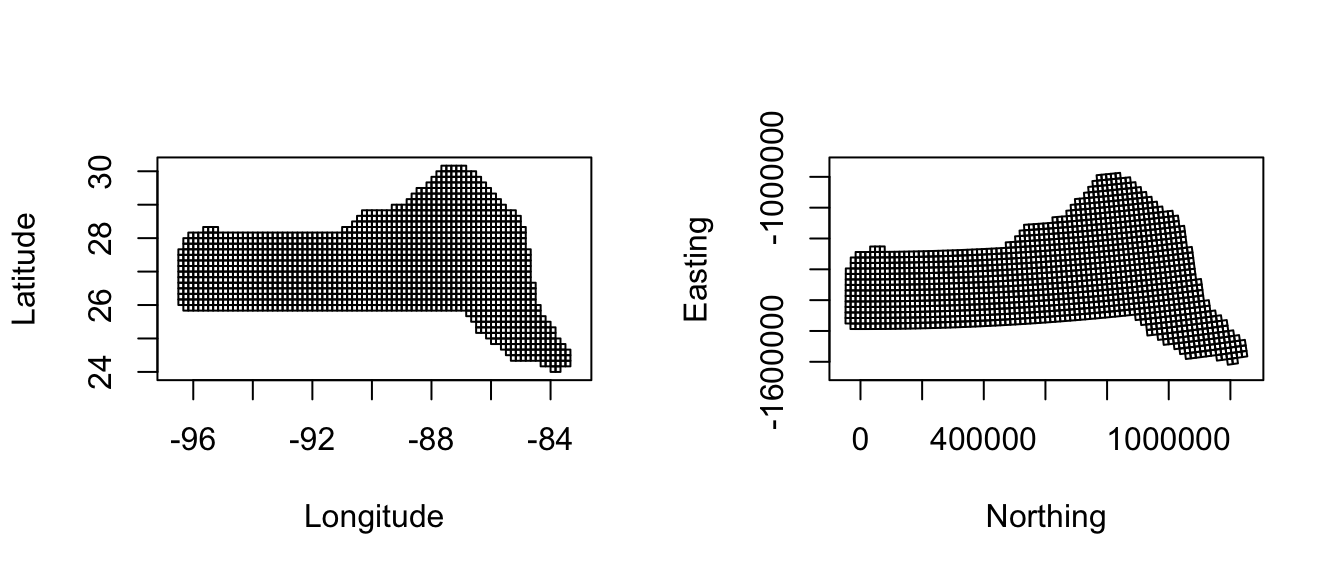# Introduction

The analysis is based on a dataset of observations of pantropical dolphins in the Gulf of Mexico (shipped with Distance 6.0 and later). For convenience the data are bundled in an `R`-friendly format, although all of the code necessary for creating the data from the Distance project files is available on github. The OBIS-SEAMAP page for the data may be found at the SEFSC GoMex Oceanic 1996 survey page.

The intention here is to highlight the features of the `dsm` package, rather than perform a full analysis of the data. For that reason, some important steps are not fully explored. Some familiarity with density surface modelling is assumed.

This is a `knitr` document. The source for this document contains everything you need to reproduce the analysis given here (aside from the data, which is included with the `dsm` package). The most recent version of this document can be found at github.com/dill/mexico-data.

The rest of this document is structured as follows: sections 1-3 deal with the data setup (including plotting considerations), section 4 begins with exploratory analysis and 5-10 consist of fitting and assessing models.

# Preamble

Before we start, we load the `dsm` package (and its dependencies) and set some options:

``library(dsm)``
``````## Loading required package: mgcv
## Loading required package: nlme
## This is mgcv 1.8-7. For overview type 'help("mgcv-package")'.
## Loading required package: mrds
## This is mrds 2.1.15
## Built: R 3.2.2; ; 2015-11-24 17:46:38 UTC; unix
## This is dsm 2.2.11
## Built: R 3.2.2; ; 2015-11-24 18:04:45 UTC; unix``````
``````library(ggplot2)

# plotting options
gg.opts <- theme(panel.grid.major=element_blank(),
panel.grid.minor=element_blank(),
panel.background=element_blank())

# make the results reproducible
set.seed(11123)``````

In order to run this vignette, you’ll need to install a few R packages. This can be done via the following call to `install.packages`:

``````install.packages(c("dsm", "Distance", "knitr", "captioner", "ggplot2", "rgdal",
"maptools", "plyr", "tweedie"))``````

# The data

## Observation and segment data

All of the data for this analysis has been nicely pre-formatted and is shipped with `dsm`. Loading that data, we can see that we have four data frames, the first few lines of each are shown:

``````data(mexdolphins)
attach(mexdolphins)``````

`segdata` holds the segment data: the transects have already been “chopped” into segments.

``head(segdata)``
``````##   longitude latitude        x        y Effort Transect.Label Sample.Label
## 1 -86.92712 29.94378 836105.9 -1011416  13800       19960417   19960417-1
## 2 -86.83176 29.84030 846012.9 -1021407  14000       19960417   19960417-2
## 3 -86.74445 29.75279 855022.6 -1029785  14000       19960417   19960417-3
## 4 -86.65230 29.65522 864610.3 -1039168  13900       19960417   19960417-4
## 5 -86.56648 29.56088 873598.1 -1048266  13800       19960417   19960417-5
## 6 -86.49290 29.49000 881203.7 -1055004  13800       19960417   19960417-6
##   depth
## 1 135.0
## 2 147.7
## 3 152.1
## 4 163.8
## 5 179.7
## 6 188.5``````

`distdata` holds the distance sampling data that will be used to fit the detection function.

``head(distdata)``
``````##     object size  distance Effort detected beaufort latitude longitude
## 45      45   21 3296.6363  36300        1        4 27.72872 -86.00159
## 61      61  150  929.1937  17800        1        4 25.99896 -87.62712
## 63      63  125 6051.0009  21000        1        2 26.00693 -87.94881
## 85      85   75 5499.6971  21800        1        1 27.50344 -90.44891
## 114    114   50 7258.9837  13400        1        3 27.40568 -94.99483
## 120    120   45 1454.7962  20900        1        5 26.01765 -95.97449
##              x        y
## 45  948000.065 -1236192
## 61  812161.653 -1436899
## 63  780969.520 -1438985
## 85  528656.807 -1297833
## 114  95910.149 -1324562
## 120   2477.665 -1473909``````

`obsdata` links the distance data to the segments.

``head(obsdata)``
``````##     object Sample.Label size  distance Effort
## 45      45   19960421-9   21 3296.6363  36300
## 61      61   19960423-7  150  929.1937  17800
## 63      63   19960423-9  125 6051.0009  21000
## 85      85   19960427-1   75 5499.6971  21800
## 114    114   19960430-8   50 7258.9837  13400
## 120    120   19960501-5   45 1454.7962  20900``````

`preddata` holds the prediction grid (which includes all the necessary covariates).

``head(preddata)``
``````##   latitude longitude        x          y depth      area
## 0 30.08333 -87.58333 774402.9 -1002759.1    35 271236913
## 1 30.08333 -87.41667 789688.6 -1001264.5    30 271236913
## 2 30.08333 -87.25000 804971.3  -999740.6    27 271236913
## 3 30.08333 -87.08333 820251.1  -998187.5    22 271236913
## 4 30.08333 -86.91667 835528.0  -996605.2    46 271236913
## 5 29.91667 -87.75000 760783.1 -1021810.3    14 271236913``````

Typically (i.e. for other datasets) it will be necessary divide the transects into segments, and allocate observations to the correct segments using a GIS or other similar package1, before starting an analysis using `dsm`.

## Shapefiles and converting units

Often data in a spatial analysis comes from many different sources. It is important to ensure that the measurements to be used in the analysis are in compatible units, otherwise the resulting estimates will be incorrect or hard to interpret. Having all of our measurements in SI units from the outset removes the need for conversion later, making life much easier.

The data are already in the appropriate units (Northings and Eastings: kilometres from a centroid, projected using the North American Lambert Conformal Conic projection).

There is extensive literature about when particular projections of latitude and longitude are appropriate and we highly recommend the reader review this for their particular study area; Bivand et al (2013) is a good starting point. The other data frames have already had their measurements appropriately converted. By convention the directions are named `x` and `y`.

Using latitude and longitude when performing spatial smoothing can be problematic when certain smoother bases are used. In particular when bivariate isotropic bases are used the non-isotropic nature of latitude and longitude is inconsistent (moving one degree in one direction is not the same as moving one degree in the other).

We give an example of projecting the polygon that defines the survey area (which as simply been read into R using `readShapeSpatial` from a shapefile produced by GIS).

``````library(rgdal)
library(maptools)
library(plyr)

# tell R that the survey.area object is currently in lat/long
proj4string(survey.area) <- CRS("+proj=longlat +datum=WGS84")

# proj 4 string
# using http://spatialreference.org/ref/esri/north-america-lambert-conformal-conic/
lcc_proj4 <- CRS("+proj=lcc +lat_1=20 +lat_2=60 +lat_0=40 +lon_0=-96 +x_0=0 +y_0=0 +ellps=GRS80 +datum=NAD83 +units=m +no_defs ")

# project using LCC
survey.area <- spTransform(survey.area, CRSobj=lcc_proj4)

# simplify the object
survey.area <- data.frame(survey.area@polygons[]@Polygons[]@coords)
names(survey.area) <- c("x", "y")``````

The below code generates Figure 1, which shows the survey area with the transect lines overlaid (using data from `segdata`).

``````p <- qplot(data=survey.area, x=x, y=y, geom="polygon",fill=I("lightblue"),
ylab="y", xlab="x", alpha=I(0.7))
p <- p + coord_equal()
p <- p + geom_line(aes(x,y,group=Transect.Label),data=segdata)
p <- p + gg.opts

print(p)``````Figure 1: The survey area with transect lines.

Also note that since we’ve projected our prediction grid, the “squares” don’t look quite like squares. So for plotting we’ll use the polygons that we’ve saved, these polygons (stored in `pred.polys`) are read from a shapefile created in GIS, the object itself is of class `SpatialPolygons` from the `sp` package. This plotting method makes plotting take a little longer, but avoids gaps and overplotting. Figure 2 compares using latitude/longitude with a projection.

``````par(mfrow=c(1,2))

# put pred.polys into lat/long
pred_latlong <- spTransform(pred.polys,CRSobj=CRS("+proj=longlat +datum=WGS84"))

# plot latlong
plot(pred_latlong, xlab="Longitude", ylab="Latitude")
axis(1); axis(2); box()

# plot as projected
plot(pred.polys, xlab="Northing", ylab="Easting")
axis(1); axis(2); box()``````Figure 2: Comparison between unprojected latitude and longitude (left) and the prediction grid projected using the North American Lambert Conformal Conic projection (right)

Tips on plotting polygons are available from the `ggplot2` wiki.

Here we define a convenience function to generate an appropriate data structure for `ggplot2` to plot:

``````# given the argument fill (the covariate vector to use as the fill) and a name,
# return a geom_polygon object
# fill must be in the same order as the polygon data
grid_plot_obj <- function(fill, name, sp){

# what was the data supplied?
names(fill) <- NULL
row.names(fill) <- NULL
data <- data.frame(fill)
names(data) <- name

spdf <- SpatialPolygonsDataFrame(sp, data)
spdf@data\$id <- rownames(spdf@data)
spdf.points <- fortify(spdf, region="id")
spdf.df <- join(spdf.points, spdf@data, by="id")

# seems to store the x/y even when projected as labelled as
# "long" and "lat"
spdf.df\$x <- spdf.df\$long
spdf.df\$y <- spdf.df\$lat

geom_polygon(aes_string(x="x",y="y",fill=name, group="group"), data=spdf.df)
}``````

# Exploratory data analysis

## Distance data

The top panels of Figure 3, below, show histograms of observed distances and cluster size, while the bottom panels show the relationship between observed distance and observed cluster size, and the relationship between observed distance and Beaufort sea state. The plots show that there is some relationship between cluster size and observed distance (fewer smaller clusters seem to be seen at larger distances).

The following code generates Figure 3:

``````par(mfrow=c(2,2))

# histograms
hist(distdata\$distance,main="",xlab="Distance (m)")
hist(distdata\$size,main="",xlab="Cluster size")

# plots of distance vs. cluster size
plot(distdata\$distance, distdata\$size, main="", xlab="Distance (m)",
ylab="Group size", pch=19, cex=0.5, col=gray(0.7))

# lm fit
l.dat <- data.frame(distance=seq(0,8000,len=1000))
lo <- lm(size~distance, data=distdata)
lines(l.dat\$distance, as.vector(predict(lo,l.dat)))

plot(distdata\$distance,distdata\$beaufort, main="", xlab="Distance (m)",
ylab="Beaufort sea state", pch=19, cex=0.5, col=gray(0.7))``````